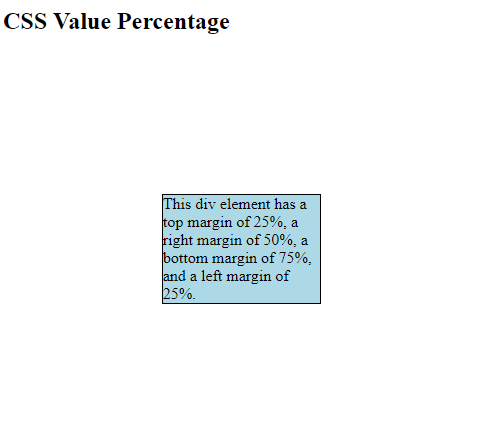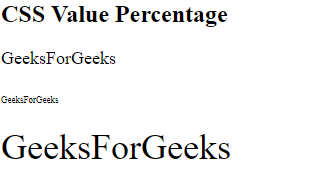Open in App
Not now

# CSS | Percentage Value

• Last Updated : 03 Jun, 2020

It represents by <percentage > takes a number as a parameter. It is used to define the relative size to the parent size. With the help of this, you can adjust all the HTML-CSS elements. In CSS, many properties that take a percentage as parameters such as padding, width, height, margin, and font-size.

Syntax:

`<Percentage>`

It takes a number as parameter followed by percentage sign(%).

The number can be positive and negative but some properties do not accept negative percentages.

Note: No space between number and percentage sign(%) is acceptable.

Example 1:

 ` ` `<``html``> ` ` `  `<``head``> ` `    ``<``style``> ` `        ``div { ` `            ``border: 1px solid black; ` `            ``margin: 25% 50% 75% 25%; ` `            ``background-color: lightblue; ` `        ``} ` `    `` ` ` ` ` `  `<``body``> ` `    ``<``h2``>CSS Value Percentage ` ` `  `    ``<``div``> ` `        ``This div element has a top margin ` `        ``of 25%, a right margin of 50%, a  ` `        ``bottom margin of 75%, and a left  ` `        ``margin of 25%. ` `    `` ` `    ``<``hr` `/> ` ` ` ` `  ` `

Output:Example 2:

 ` ` `<``html``> ` ` `  `<``head``> ` `    ``<``title``> ` `        ``CSS | Percentage Value ` `    `` ` ` ` ` `  `<``body``> ` `    ``<``h2``>CSS Value Percentage ` ` `  `    ``` `    ``<``div` `style``=``"font-size: 18px;"``> ` ` `  `        ``<``p``>GeeksForGeeks ` ` `  `        ``` `        ``<``p``><``span` `style``=``"font-size: 50%;"``> ` `                ``GeeksForGeeks ` `            `` ` `        `` ` ` `  `        ``` `        ``<``p``><``span` `style``=``"font-size: 200%;"``> ` `                ``GeeksForGeeks ` `            `` ` `        `` ` `    `` ` ` `  `    ``<``hr` `/> ` ` ` ` `  ` `

Output:My Personal Notes arrow_drop_up
Related Articles